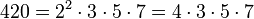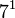# Groups of order 420

## Contents

See pages on algebraic structures of order 420| See pages on groups of a particular order

## Statistics at a glance

The number 420 has the prime factorization:$\! 420 = 2^2 \cdot 3 \cdot 5 \cdot 7 = 4 \cdot 3 \cdot 5 \cdot 7$

Quantity Value List/comment
Total number of groups 41
Number of abelian groups 2 (number of abelian groups of order$2^2$) times ((number of abelian groups of order$3^1$) times (number of abelian groups of order$5^1$) times (number of abelian groups of order$7^1$) = (number of unordered integer partitions of 2) times (number of unordered integer partitions of 1) times (number of unordered integer partitions of 1) times (number of unordered integer partitions of 1) =$2 \times 1 \times 1 \times 1 = 2$. See classification of finite abelian groups and structure theorem for finitely generated abelian groups.
Number of nilpotent groups 2 ((number of groups of order 4) times (number of groups of order 3) times (number of groups of order 5) times (number of groups of order 7) =$2 \times 1 \times 1 \times 1 = 2$. See number of nilpotent groups equals product of number of groups of order each maximal prime power divisor, which in turn follows from equivalence of definitions of finite nilpotent group.
Number of solvable groups 40 The only non-solvable group is direct product of A5 and Z7 (ID: (420, 13)). Note that this group is 7-solvable (i.e., 7-separable) and hence also$\{ 2,3,5 \}$-separable. See p-solvable group and pi-separable group.
Number of simple groups 0 The only non-solvable group is a direct product of smaller groups.

## GAP implementation

The order 420 is part of GAP's SmallGroup library. Hence, any group of order 420 can be constructed using the SmallGroup function by specifying its group ID. Also, IdGroup is available, so the group ID of any group of this order can be queried.

Further, the collection of all groups of order 420 can be accessed as a list using GAP's AllSmallGroups function.

Here is GAP's summary information about how it stores groups of this order, accessed using GAP's SmallGroupsInformation function:

gap> SmallGroupsInformation(420);

There are 41 groups of order 420.
They are sorted by their Frattini factors.
1 has Frattini factor [ 210, 1 ].
2 has Frattini factor [ 210, 2 ].
3 has Frattini factor [ 210, 3 ].
4 has Frattini factor [ 210, 4 ].
5 has Frattini factor [ 210, 5 ].
6 has Frattini factor [ 210, 6 ].
7 has Frattini factor [ 210, 7 ].
8 has Frattini factor [ 210, 8 ].
9 has Frattini factor [ 210, 9 ].
10 has Frattini factor [ 210, 10 ].
11 has Frattini factor [ 210, 11 ].
12 has Frattini factor [ 210, 12 ].
13 - 41 have trivial Frattini subgroup.

For the selection functions the values of the following attributes
are precomputed and stored:
IsAbelian, IsNilpotentGroup, IsSupersolvableGroup, IsSolvableGroup,
LGLength, FrattinifactorSize and FrattinifactorId.

This size belongs to layer 2 of the SmallGroups library.
IdSmallGroup is available for this size.